# Inductive Reasoning ExplanationOn this page we will discuss the most common patterns for inductive reasoning questions. You can choose to either first study this page and then start practising, or first start practising and study this page when you find exercises that you don’t understand. It differs per person what works best.

Inductive reasoning, also known as abstract reasoning or figure sequences, can be roughly divided into two types of questions:

1. Dynamic series. The exercise consists of a number of images in which something changes according to a certain rule or several rules. The task is to find the rules/patterns in the given images and to select the image that completes the series.
2. Static series. The exercise again consists of a number of images, but now you have to look for the similarities between all images. For the correct answer, the image must be selected that meets the same characteristics / rules.

Example dynamic series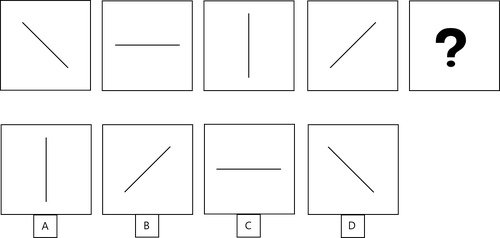This is a dynamic series. The figure rotates with 45 degrees counterclockwise from the first to the second image and then rotates with 90 degrees from the 2nd to the 3rd. This is repeated. You can find the correct answer by rotating the line in figure 4 with 90 degrees. The correct answer is D.

Example static sequence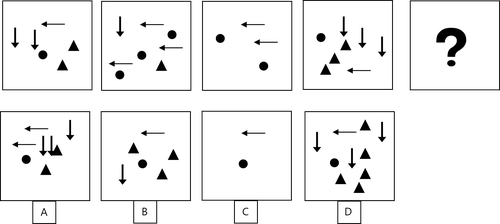This is a static sequence. The number of arrows pointing to the left is always equal to the number of circles and the number of arrows pointing down is always equal to the number of triangles. The answer has to be C.

It can sometimes be difficult to see whether a question contains a static or a dynamic sequence. By reading the explanations for all types of series carefully and practicing a lot, you will work faster, make fewer mistakes and get a better result on your assessment.

Dynamic series

Most series can be solved by finding out the rule that is applied to find the next figure in the series. The number of possible rules is unlimited, so you will need to look at some of the exercises with an open mind. However, in practice the vast majority of the rules/patterns are based on four types of rules:

1. Increase or decrease of the number of figures.
2. Change of the angle of the figures.
3. Movement of the figures within the image
4. Change of color or other characteristics of the figures

Often a combination of these types of rules are applied in exercises . Sometimes you are deliberately put on the wrong track with irrelevant information in the figures that is not based on a clear rule, for example a figure that rotates randomly (see the first example below).

Increase or decrease of the number of figures.

Check for this pattern if you see multiple elements in all images. For example multiple arrows, figures with a different number of dots, a different total number of figures per image, etc.

Example: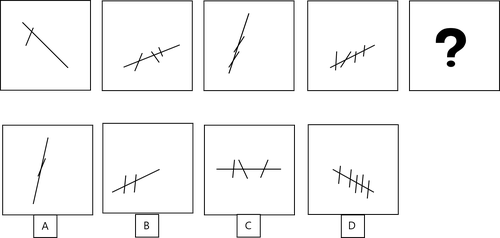The whole figure rotates, but there does not seem to be a clear pattern in the angles. In every figure there is a straight line, with one or more shorter transverse lines. The first figure has 1 transverse line, then 3, then 2, then 4. Two transverse lines are added and then one disappears again, this is repeated. The answer must have 3 transverse lines and the only correct option is C.

Change of the angle of the figures.

With this pattern there will always be figures that have a certain angle in the image as a whole, or relative to each other. Check for this pattern if you see figures that rotate in the different images.

Example: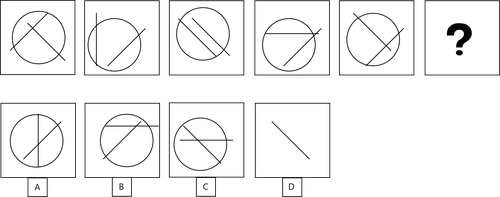In every image there are two straight lines of equal length that make an angle. If you look closely, you can see that there is a line that rotates 45 degrees counterclockwise each step (the line at the top left of the first image). The other line rotates with 90 degrees each time (since the line is symmetrical, the figure moves from 45 degrees one way to 45 degrees the other way). The answer is A.

Movement of the figures within the image

In this pattern, generally several figures move through the image. Sometimes the same figure is included twice to make it more difficult to see the pattern.

Example: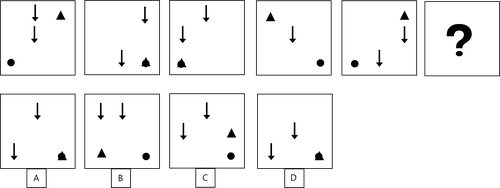There are 4 figures that move through the image. The circle moves from the left lower corner to the lower right corner and then back again. The triangle rotates clockwise to the corners of the image. One of the arrows moves vertically through the center of the image and starts again at the top when the end of the image is reached. The other arrow moves horizontally and moves down one row when the end of the image is reached. You can see that answer A is the only figure that meets all these rules.

Color or other features of the figures

This pattern will often be combined with the other basic patterns. For example, you could see this pattern combined with the previous example:

Example: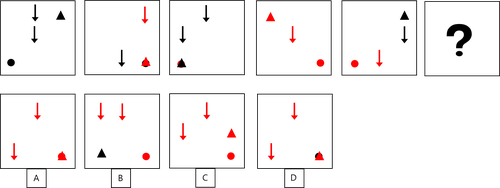You can see that the same rules apply as in the previous example:

• The circle moves back and forth between the left and right bottom corners
• The triangle moves clockwise along all corners of the image
• The top arrow in the first figure moves one place to the right each time. When the end of the figure is reached, the figure moves down a row and starts all the way to the left.
• The bottom arrow in the first figure keeps moving down and starts again at the top when the bottom of the image is reached.

In addition, the first image contains 0 red figures, then 2, then 1, then 3, then 2. Alternately two figures turn red, and then 1 black again. The answer must contain 4 red figures. The correct answer is A.

Many of the exercises in your assessment, especially the more difficult ones, are based on several of the above patterns. It can be useful to write down every pattern you can deduct from the images. This way you can quickly eliminate possible answers that do not meet the rules.

Our practice assignments cover all types of patterns that you will encounter in your assessment. All details have been elaborated in detail, so that you know exactly how to solve the problems.

You can take the following tips into account:

1. Apply a pattern immediately if you recognize it. Don’t try to find all the rules before you start working towards the answer.
2. If you find a pattern, write it down immediately. It is very easy to lose oversight if you don’t make notes.
3. Immediately eliminate the answers that do not match the patterns you have already found. This makes it easier to test the next pattern, because you don’t have to look at all the answers anymore.
4. Don't linger too long on one assignment. Some exercises are very difficult and it’s not strange if you miss one or two. It is a shame if you can’t finish the assessment because you have been stuck with 1 question for a long time. Sometimes you just don't see it and you have to make an (educated) guess.

Static series

With every exercise, your first reaction will be to find the rule that can bring you the next image in the sequence. However, you will always have to take into account that the sequence can be static. In this case you have to look for patterns in the similarities between the images, instead of patterns in the changes between the exercises.

The assignment is almost always to derive an underlying rule or principle from a number of given images; the common denominator in all images. Usually you will be asked to select the figure that fits within the series, but you may also have to indicate which alternative does not belong in a series of images. Both assignments come down to the same thing, because you will have to find the common denominator for both assignments.

Example: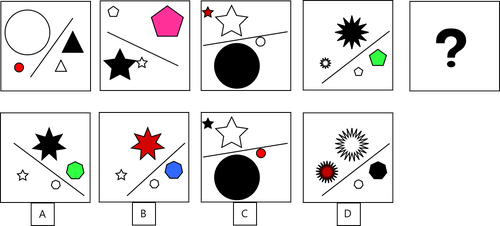If you look for a dynamic rule, you probably won't find a suitable pattern. You have to look for similarities between the different images. You can find (among others) the following similar characteristics.

• All images contain 4 figures and 1 straight line. Unfortunately, this also applies to all possible answers.
• All figures contain 2 times a large and a small figure that are equal to each other. Unfortunately, this is also the case with all possible answers.
• All images have exactly 1 black colored figure. Answers B and C are therefore excluded.
• You can see that 2 equal figures are separated from the 2 other equal figures by a straight line. Answer A falls out because the line separates the similar images.
• The answer must be D.

You have to pay attention to many different aspects of the figures to find the answer. In general, many of the following types of characteristics are used in the exercises:

1. The total number of figures in the image
2. Similarities between figures in the different images. For example:
1. color
2. striped / normally
3. open / closed figure
4. number of angles
5. straight / curved lines
6. right angles / wide angles
7. large / small figures
3. The dimensions of the figures
4. The angle the figures make
5. The position of the figures in the image
6. Figures relative to each other (perpendicular to each other, making a right angle, parallel, always between two other figures, etc.)

In general, several of the above options need to be found in an exercise. With static series it is even more important to be very precise than with dynamic series. If you have found a pattern, you should immediately eliminate the answers that do not match the rule. This way you can quickly search for the next pattern, until you have found the correct answer.

You can take the following tips into account:

1. Look for concrete agreements that you can clearly identify. Do not work too intuitively, the assignments are often designed to mislead your feelings.
2. Work from easy to difficult. For example, always first count the number of figures in the image. You might already be able to eliminate an answer.
3. Write down all the patterns you've already tested so you don't do double work or lose oversight. You can design a system for yourself that makes it easy to write down the patterns that you find.
1. Write down the number of figures per image with a number.
2. Write down the applicable characteristics, you can make up abbreviations for yourself. RAfor a right angle, S if the size of the images is relevant, etc.
3. Write down the answers that are eliminated, so ABD for example means that only have to look at C and E for the next pattern.

Determine which strategy works best for you with the practice exercises. This way you stay calmer during your assessment and you will not be faced with surprises.

Our practice assignments cover all types of patterns that you will also encounter in your assessment. All exercises have worked out solutions.